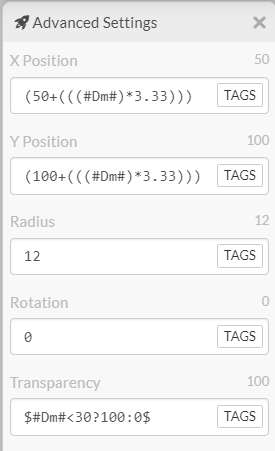# [ SOLVED ] Linear Angular Movement Per Half Hour

I’ve got a watchface I’m working on that has a ball moving at a -45 degree angle between 0 and 30 minutes

At which point, I’ll have another ball moving at 315 from 30 minutes to the top of the hour.

I’m avoiding using a sin/cos as I don’t want a loop animation.

Something like this:

Thanks in advance for any help!

If it is a point A to Point B question, then that should be relatively easy. For green, x and y both increase at a fixed rate between those points. For red, x and y go negative (at the same rate).

For example sake lets say they move on an equal 45 degree angle.

Green element:
start x = 50
end x = 150
Total movement = 100

start y = 100
end y = 200
Total movement = 100

And we want to move 100 pixels over the course of 30 minutes (#Dm#). So 100/30 = `((#Dm#)*3.33)` To this you need to add a starting point.

The issue then becomes what to do when reversing direction. The element will continue to move in that direction for the full hour. You just need to add a transparency for the time it should be hidden. A conditional should take care of that:
`\$#Dm#<30?100:0\$` for element 1.Element 2 is a little trickier since the element will be transparent during the first half of the hour. You will need to set the starting point double the distance away so it becomes visible where you want it to start.1 Like

I think I get it now…

First you have to get the distance travelled and divide it by number of minutes… that makes sense!

Thanks so much! I was starting to get desperate and use growing bars instead of a moving object.

1 Like